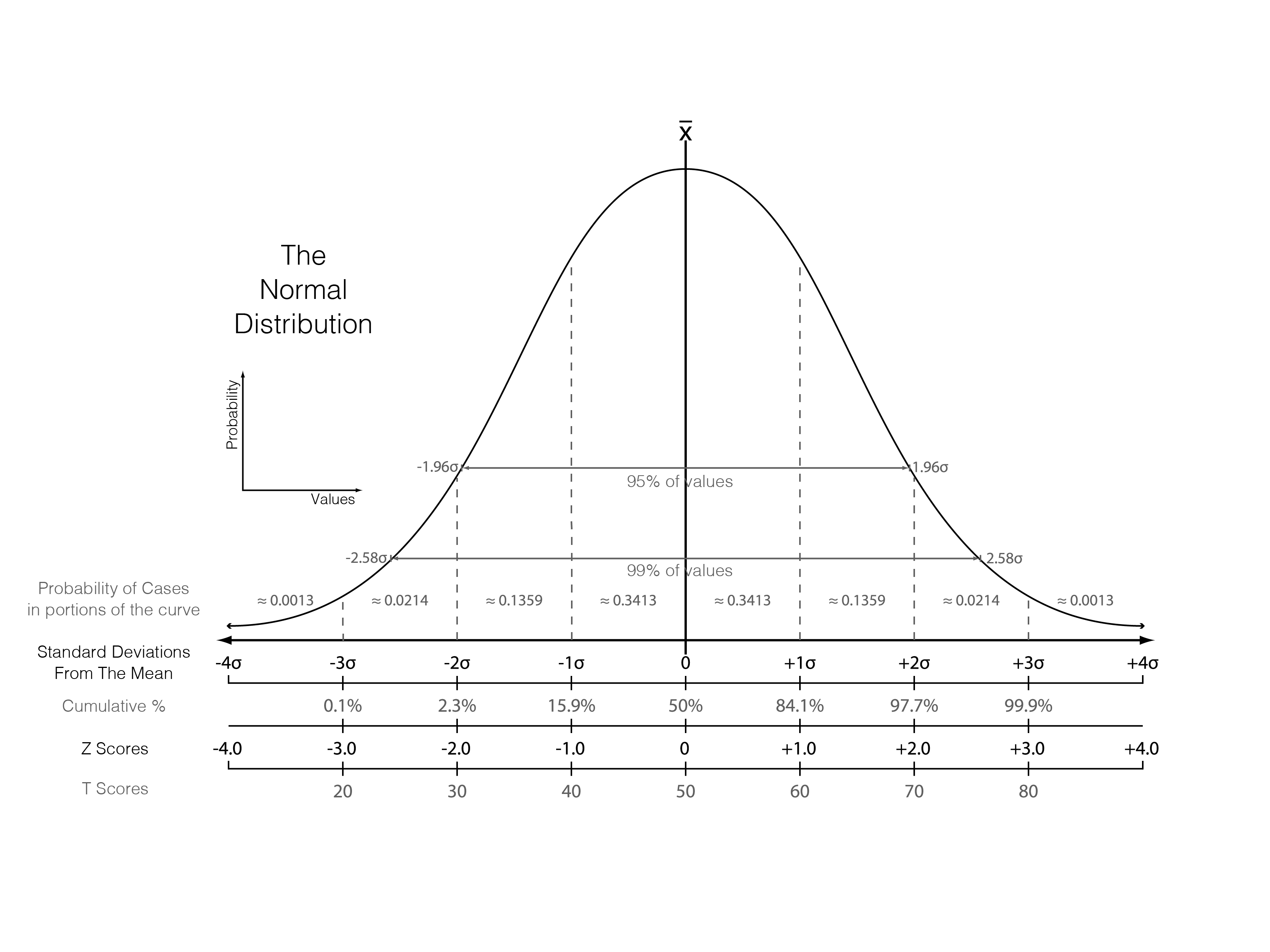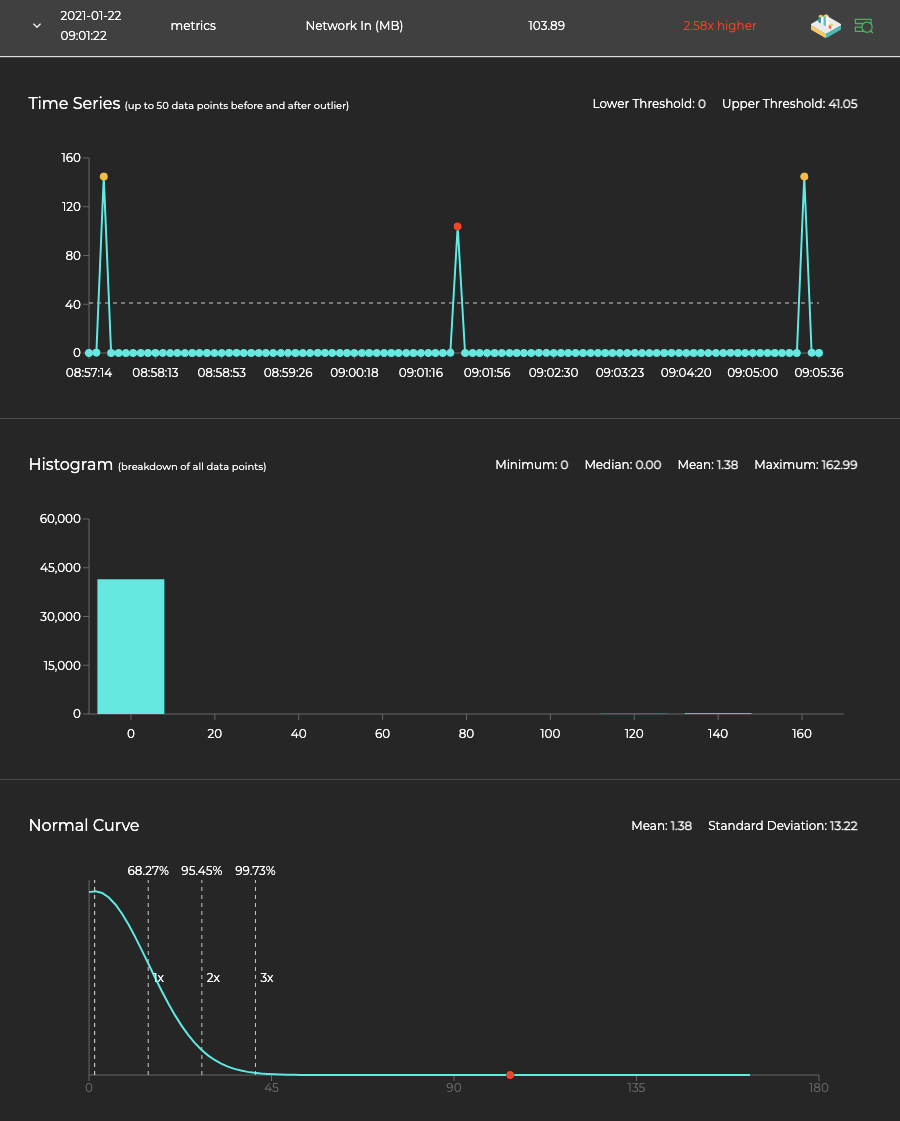# Method for Outlier Detection: Standard Deviation - Standard

The standard score is the number of standard deviations by which the data point is above or below the mean value. The following diagram shows the standard deviations from the mean.A point beyond 3 standard deviations from the mean on either side is considered an outlier. In the below standard deviation curve, the outlier data point is 2.58 times the 3 standard deviation threshold away from the mean: